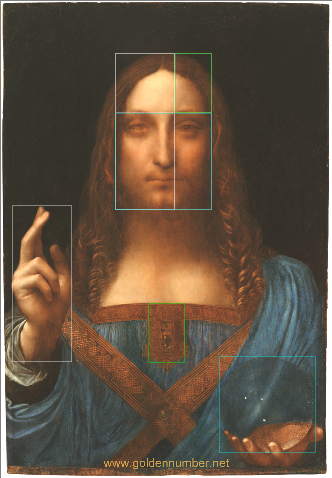# What is the Golden Ratio?

What is the golden ratio? First off let’s look at the definition of the word ratio:

“the quantitative relation between two amounts showing the number of times one value contains or is contained within the other”

The golden ratio is 1:1.618

If you want to know the history of how it was found check out this article by Esther Inglis-Arkel.

Next we need  to look at how the golden ratio is related to the Fibonacci sequence. The Fibonacci sequence is a range of numbers where each number is the sum of the 2 numbers preceding it:

0, 1, 1, 2, 3, 5, 8, 13, 21, 34, 55 and so on.

It is linked with the golden ratio because as you go along the sequence and divide each number by the preceding number you always arrive at 1.6. i.e. the golden ratio.

This discovery created huge excitement and it became known as ‘the divine proportion’. Suddenly everywhere people looked it appeared: it seems we are hardwired to be attracted to things using the Fibonacci sequence or golden ratio.  in nature:In the helixes, seed patterns and unfurling of ferns. Even the helix of our DNA follows a Fibonacci sequence.

In architecture:Image: Gizmodo

The divine proportion was used to make what became known as the golden rectangle. The most beautiful mathematical shape. This was then used as a way to work out the proportions of buildings when designing them.

Golden Ratio in Art:Image by Gary Meisner (2014): The Golden Number

By setting out the canvas using ‘the golden rectangle’ the artist was able to create an innate sense of balance and harmony in the work.

There are many examples of how Artists both past and present use the divine proportion or golden ratio in art.

When we feel that a piece is complete or fully resolved it is often because the golden ratio and principles of sacred geometry have been used.